The area of a convex hull enables you to estimate the area of a compact region from a set of discrete observations. For example, a biologist might have multiple sightings of a wolf pack and want to use the convex hull to estimate the area of the wolves' territory. A forestry expert might use a convex hull to estimate the area of a forest that has been infested by a beetle or a blight. This article shows how to compute the area and perimeters of convex hulls in SAS. Because a convex hull is a convex polygon, we present formulas for the area and perimeter of polygons and apply those formulas to convex hulls.

### Gauss' shoelace formula for the area of a polygon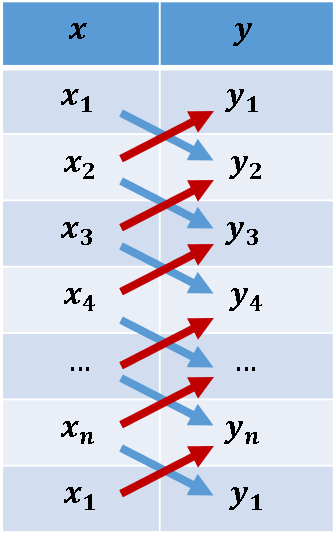There are many formulas for the area of a planar polygon, but the one used in this article is known as Gauss' shoelace formula, or the triangle formula. This formula enables you to compute the area of a polygon by traversing the vertices of the polygon in order. The formula uses only the coordinates of adjacent pairs of vertices. Assume a polygon has n that are vertices enumerated counterclockwise, and the coordinates are $(x_1, y_1), (x_2, y_2), \ldots, (x_n, y_n)$. Then the area of the polygon is given by the shoelace formula
$A = \frac{1}{2} \sum_{i=1}^{n} x_i y_{i+1} - x_{i+1} y_i$
where we use the convention that $(x_{n+1}, y_{n+1}) = (x_1, y_1)$.

To me, the remarkable thing about this formula is that it looks like it has nothing to do with an area. However, the Appendix derives Gauss' shoelace formula from Green's theorem in the plane, which reveals the connection between the formula and the area of the polygon.

You might wonder why this is called the "shoelace formula." Suppose a polygon has vertices $(x_1, y_1), (x_2, y_2), \ldots, (x_n, y_n)$. A schematic visualization of the formula is shown to the right. You list the X and Y coordinates of the vertices and append the first vertex to the end of the list. The blue arrows represent multiplication. The red arrows represent multiplication with a negative sign. So, the first two rows indicate the quantity $x_1 y_2 - x_2 y_1$. (If you are familiar with linear algebra, you can recognize this quantity as the determinant of the 2 x 2 matrix with first row $(x_1, y_1)$ and second row $(x_2, y_2)$.) The sequence of arrows looks like criss-cross lacing on a shoe.

### Implementing the area and perimeter of a polygon in SAS

The visualization of the shoelace formula suggests an efficient method for computing the area of a polygon. First, append the first vertex to the end of the list of vertices. Then, define the vector Lx = lag(x) as the vector with elements $x_2, x_3, \ldots, x_n, x_1$. Define Ly = lag(y) similarly. Then form the vector d = x#Ly – y#Lx, where the '#' operator indicates elementwise multiplication. The area of the polygon is the sum(d)/2. This computation is implemented in the following SAS/IML program, which also implements a function that computes the Euclidean distance along the edges of the polygon. The perimeter formula is given by
$s = \sum_{i=1}^{n} \sqrt{(x_{i+1} -x_i)^2 + (y_{i+1} -y_i)^2}$, where $(x_{n+1}, y_{n+1}) = (x_1, y_1)$.

proc iml; /* The following functions are modified from Rick Wicklin (2016). The Polygon package. SAS Global Forum 2016 */ /* PolyArea: Return the area of a simple polygon. P is an N x 2 matrix of (x,y) values for vertices. N > 2. This function uses Gauss' "shoelace formula," which is also known as the "triangle formula." See https://en.wikipedia.org/wiki/Shoelace_formula */ start PolyArea(P); lagIdx = 2:nrow(P) || 1; /* vertices of the lag; appends first vertex to the end of P */ xi = P[ ,1]; yi = P[ ,2]; xip1 = xi[lagIdx]; yip1 = yi[lagIdx]; area = 0.5 * sum(xi#yip1 - xip1#yi); return( area ); finish;   /* PolyPerimeter: Return the perimeter of a polygon. P is an N x 2 matrix of (x,y) values for vertices. N > 2. */ start PolyPeri(_P); P = _P // _P[1,]; /* append first vertex */ /* (x_{i+1} - x_i)##2 + (y_{i+1} - y_i)##2 */ v = dif(P[,1])##2 + dif(P[,2])##2; /* squared distance from i_th to (i+1)st vertex */ peri = sum(sqrt(v)); /* sum of edge lengths */ return( peri ); finish;   /* demonstrate by using a 3:4:5 right triangle */ Pts = {1 1, 4 1, 4 5}; Area = PolyArea(Pts); Peri = PolyPeri(Pts); print Area Peri;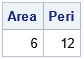The output shows the area and perimeter for a 3:4:5 right triangle with vertices (1,1), (4,1), and (4,5). As expected, the area is 0.5*base*height = 0.5*3*4 = 6. The perimeter is the sum of the edge lengths: 3+4+5 = 12.

Notice that neither of the functions uses a loop over the vertices of the polygon. Instead, the area is computed by using vectorized computations. Essentially, the area formula uses the LAG of the vertex coordinates, and the perimeter formula uses the DIF of the coordinates.

### Apply the area and perimeter formulas to convex hulls

You can use the previous functions to compute the area and perimeter of the 2-D convex hull of a set of planar points. In SAS, you can use the CVEXHULL function to compute a convex hull. The CVEXHULL function returns the indices of the points on the convex hull in counterclockwise order. You can therefore extract those points to obtain the polygon that encloses the points. You can send this polygon to the functions in the previous section to obtain the area and perimeter of the convex hull, as follows:

/* Use the CVEXHULL function in SAS to compute 2-D convex hulls. See https://blogs.sas.com/content/iml/2021/11/15/2d-convex-hull-sas.html */ points = {0 2, 0.5 2, 1 2, 0.5 1, 0 0, 0.5 0, 1 0, 2 -1, 2 0, 2 1, 3 0, 4 1, 4 0, 4 -1, 5 2, 5 1, 5 0, 6 0 };   /* Find the convex hull: indices on the convex hull are positive */ Indices = cvexhull( points ); hullIdx = indices[loc(indices>0)]; /* extract vertices with positive indices */ convexPoly = points[hullIdx, ]; /* extract rows to form a polygon */ print hullIdx convexPoly[c={'cx' 'cy'} L=""];   /* what is the area of the convex hull? */ AreaCH = PolyArea(convexPoly); PeriCH = PolyPeri(convexPoly); print AreaCH PeriCH;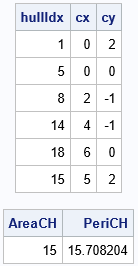The output shows the indices and coordinates of the convex polygon that bounds the set of points. It is a two-column matrix, where each row represents the coordinates of a vertex of the polygon. The area of this polygon is 15 square units. The perimeter is 15.71 units. If these coordinates are the locations of spatial observations, you can compute the area and perimeter of the convex hull that contains the points.

### Summary

A convex hull is a polygon. Accordingly, you can use formulas for the area and perimeter of a polygon to obtain the area and perimeter of a convex hull. This article shows how to efficiently compute the area and perimeter of the convex hull of a set of planar points.

### Appendix: Derive Gauss' shoelace formula from Green's theorem

If a polygon has n vertices with coordinates $(x_1, y_1), (x_2, y_2), \ldots, (x_n, y_n)$, we want to prove Gauss' shoelace formula:
$A = \frac{1}{2} \sum_{i=1}^{n} x_i y_{i+1} - x_{i+1} y_i$
where we use the convention that $(x_{n+1}, y_{n+1}) = (x_1, y_1)$.

At first glance, Gauss' shoelace formula does not appear to be related to areas. However, the formula is connected to area because of a result from multivariable calculus known as "the area corollary of Green's theorem in the plane." Green's theorem enables you to compute the area of a planar region by computing a line integral around the boundary of the region. That is what is happening in Gauss' shoelace formula. The "area corollary" says that you can compute the area of the polygon by computing $\oint_C x/2\; dx - y/2\; dy$, where C is the piecewise linear path along the perimeter of the polygon.

Let's evaluate the integral on the edge between the i_th and (i+1)st vertex. Parameterize the i_th edge as
$(x(t), y(t)) = ( x_i + (x_{i+1}-x_i)t, y_i + (y_{i+1}-y_i)t )\quad \mathrm{for} \quad t \in [0,1]$. With this parameterization, $dx = (dx/dt)\; dt = (x_{i+1}-x_i)\; dt$ and $dy = (dy/dt)\; dt = (y_{i+1}-y_i)\; dt$.

When you substitute these expressions into the line integral, the integrand becomes a linear polynomial in t, but a little algebra shows that the linear terms cancel and only the constant terms remain. Therefore, the line integral on the i_th edge becomes
$\frac{1}{2} \int_{\mathrm edge} x\; dx - y\; dy = \frac{1}{2} \int_0^1 x_i (y_{i+1}-y_i) - y_i (x_{i+1}-x_i) dt = \frac{1}{2} (x_i y_{i+1}- y_i x_{i+1})$.
When you evaluate the integral on all edges of the polygon, you get a summation, which proves Gauss' shoelace formula for the area of a polygon.

The post The area and perimeter of a convex hull appeared first on The DO Loop.

On this Pi Day, let's explore the "πth roots of unity." (Pi Day is celebrated in the US on 3/14 to celebrate π ≈ 3.14159....) It's okay if you've never heard of the πth roots of unity. This article starts by reviewing the better-known nth roots of unity. It then shows all the math you'll need to understand the πth roots of unity and how to visualize them!

### The nth roots of unity

Recall that the nth root of unity for a positive integer, n, is a complex number, z, such that the nth power of z equals 1. In symbols, z satisfies the equation zn = 1.

For any positive integer, n, there are n roots of unity. The nth roots of unity are the complex numbers
$\exp(2\pi i k /n) = \cos(2 \pi k/n) + i \sin(2 \pi k/n)$
for k=0, 1, 2, ..., n. Geometrically, these points are the equally spaced points on the unit circle. You get them by tracing the image of the point 1 + 0*i as you rotate the circle by 2π/n radians. Some simple examples are:

• n=2. The square roots of unity are the numbers 1 and -1. Geometrically, these points are represented in the Argand plane by the ordered pairs (1, 0) and (-1, 0). They are the orbit of the point (1,0) under rotations by π radians.
• n=3. The cube roots of unity are the numbers 1, -1/2 + i sqrt(3)/2, and -1/2 - i sqrt(3)/2. These points are the orbit of the point (1,0) under rotations by 2π/3 radians.
• n=4. The 4th roots of unity are the numbers 1, i, -1, and -i. The coordinates of these points on the unit circle are (1,0), (0, 1), (-1, 0), and (0, -1). These points are the orbit of the point (1,0) under rotations by π/2 radians.

### The πth roots of unity

So, what is a πth root of unity? It is complex number, z, such that zπ = 1. Geometrically, it is one of the points on the orbit of the point (1,0) by rotations of 2*pi/pi = 2 radians. These complex numbers have the formula $z = \exp(2 i n)$ for any integer, n. You can verify that these numbers are the πth roots of unity by showing that $z^\pi = 1$, as follows:
$\exp(2 i n)^\pi = \exp(2\pi i n) = \cos(2 \pi n) + i \sin(2 \pi n) = 1$ for all integers n.

Notice that the "circle map" x → exp(i x) sends the even integers to the πth roots of unity. Thus, you can also refer to the points as the image of the even integers under the mapping.

Geometrically, the πth roots are generated by rotating the unit circle by 2 radians or by -2 radians. For simplicity, the subsequent sections visualize the πth roots for only the positive integers, which correspond to counterclockwise rotations by 2 radians.

### Visualize the πth roots of unity

Let's generate some positive integers and plot the complex points exp(2 i n) in the Argand plane. To get started, let's plot only the images of the first 25 even integers:

%let maxN = 355; data Roots; do n = 1 to &maxN; s = 2*n; xn = cos(s); /* image of even integers under mapping to unit circle */ yn = sin(s); output; end; run;   /* define a helpful macro */ %macro PlotCircle(); ellipseparm semimajor=1 semiminor=1 / xorigin=0 yorigin=0 outline lineattrs=(color=cxCCCCCC); %mend;   ods graphics / width=400px height=400px; title "Wrap Positive Even Integers onto a Circle"; title2 "First 25 Even Integers"; proc sgplot data=Roots(obs=25) aspect=1 noautolegend; %PlotCircle; scatter x=xn y=yn / datalabel=s; refline 0 / axis=x; refline 0 / axis=y; xaxis display=none; yaxis display=none; run;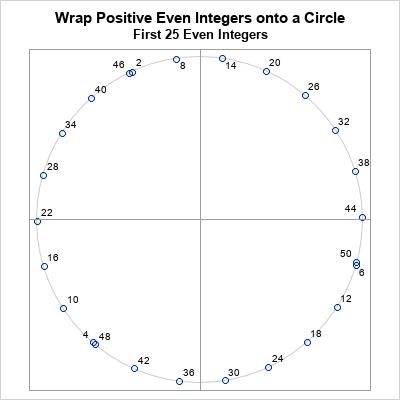Geometrically, you can think of wrapping the real number line onto the circle like thread onto a spool. The points show the images of the even integers. You can see that 2 is mapped into the second quadrant, 4 is mapped to the third quadrant, and 6 is mapped to the fourth quadrant just below the point (1,0) because 6 radians is slightly less than 2π. This pattern repeats for the images of 8, 10, and 12. Each set of three points slightly lags the previous set of three points.

The image of 22 is very close to (-1, 0). This is because 22/7 is a close approximation of π, so 22 ≈ 7π. Similarly, the image of 44 ≈ 14π is close to the point (1, 0).

The first 22 images are nearly equally spaced on the circle, but not quite. You can see that the images of 46, 48, and 50 almost overlay the images of 2, 4, and 6, but are slightly separated. This pattern continues for the next set of 22 even integers, and the next, and the next. No image ever hits a previous image because we are rotating by 2 radians and there are 2π radians in a circle. (These numbers are not commensurable.) The following graph shows the image of a few hundred even integers onto the circle: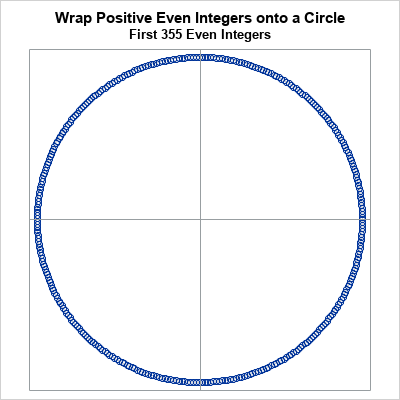This graph visualizes the πth roots of unity. The images of all positive and all negative integers will never overlap or repeat. By the irrational rotation theorem (rescaled), the πth roots of unity are dense in the circle.

### The Beatty sequence on the circle

In a previous article, I showed that every positive integer belongs to one of two disjoint sets. Each positive integer belongs to the Beatty sequence for π, or it belongs to the complementary sequence. The same is true for the even positive integers: some are elements of the Beatty sequence for π, and the rest are not. Let's see what happens if we color each point on the circle according to whether it is the image of the Beatty sequence: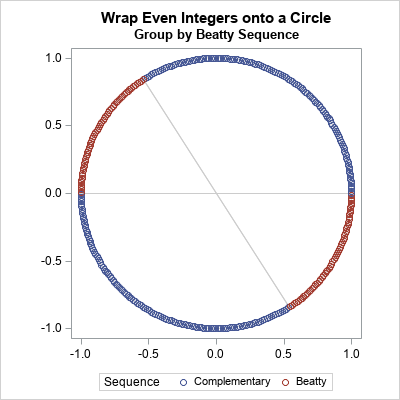Did you expect this result? Wow! The graph shows that all of the integers that belong to the Beatty sequence are mapped into two arcs on the unit circle. The red arc in the second quadrant is for radian angles in the interval (π-1, π). The red arc in the fourth quadrant is for radian angles in the interval (2π-1, 2π). These values can be deduced by using the definition of the Beatty sequence, and the fact that a Beatty number can be represented as floor(k π) = k π - f for some fractional part f in the interval (0, 1).

The graph shows that (1/π)th of the πth roots of unity belong to the Beatty sequence for π. And these numbers are all clumped together into two arcs on the circle.

Technically, the Beatty sequence is defined only for positive integers. Thus, I've illustrated this result for positive integers. However, you can extend the Beatty sequence to all non-zero integers in a natural way. If you do that, then the roots of unity that belong to the "negative Beatty sequence" are visualized by reflecting the circle across the horizontal axis. If you overlay the two circles, the extended red and blue markers interlace, and the result is not as striking.

### Summary

There are infinitely many πth roots of unity. They are complex numbers of the form $\exp(2 i n)$ for any integer, n. Geometrically, you can visualize these points as the rotations by ±2 radians of the point (1,0) in the Argand plane. The πth roots of unity are dense in the unit circle. If you restrict your attention to only positive rotations (the images of the positive integers), you can classify each root according to whether it is the image of an even integer in the Beatty sequence for π or in the complementary sequence. The points that are in the Beatty sequence are mapped to the arcs for angles (π-1, π) and (2π-1, 2π). These arcs are (1/π)th of the arclength of the unit circle.

The post The pi_th roots of unity appeared first on The DO Loop.

Did you know that you can use π to partition the positive integers into two disjoint groups? It's not hard. One group is generated by the integer portions of multiples of π. The FLOOR function gives the integer portion of a positive number, so you can write integer that are generated from π as Bn = {floor(n*π)} for n=1,2,3,.... This is called the Beatty sequence for π. The first few numbers in the Beatty sequence for π are 3, 6, 9, 12, 15, 18, 21, 25, 28, 31, 34, 37, 40, 43, 47, 50, 53, ....

The second group contains all the positive integers that are not in the Beatty sequence: 1, 2, 4, 5, 7, 8, 10, 11, 13, 14, 16, 17, 19, 20, 22, 23, 24, 26, .... A remarkable fact is that the second group of integers is also the Beatty sequence for some number! In fact, it is the Beatty sequence for the number π/(π-1). So, the positive integers are partitioned into two mutually disjoint groups by using the Beatty sequence for π and the complementary Beatty sequence for π/(π-1). These two sequences generate all positive integers, and no integer is in both sequences. For example, the number 2020 appears only in the Beatty sequence for π whereas 2022 appears in the complementary sequence.

It turns out that the only properties of π that are needed for this result is the fact that π is irrational and π > 1, a result that is known as Beatty's Theorem. This article uses SAS to illustrate the Beatty sequence for π and its complementary sequence.

### The Beatty sequence

For any irrational number r > 1, the Beatty sequence for r is integer portion of the sequence r, 2*r, 3*r, .... You can compute the Beatty sequence as Bn = {floor(n*r)} for n=1,2,3,.... The complementary sequence is generated by the irrational number c = r/(r-1) in the same way. The complementary sequence is Cn = {floor(n*c)} for n=1,2,3,....

With these definitions, Beatty's theorem (also called Rayleigh's theorem) states that for any irrational number, r > 1, the Beatty sequence and the complementary sequence are disjoint and generate all positive integers. For every positive integer, y, either y is an element of the Beatty sequence or y is an element of the complementary sequence. Thus, the Beatty sequence and its complementary sequence partition the integers into two disjoint parts.

For a formal proof, see the Wikipedia article or excellent "Cut the Knot" web site.

### The Beatty sequence for pi

Let's illustrate the theorem by using r = π and a finite number of positive integers. The following example uses the first 355 terms of the Beatty and complementary sequences, which cover the first 520 positive integers. Why 355 terms? Because 355/113 is part of the continued fraction expansion of π, and is an excellent approximation of π.

First, let's visualize the individual sequences. Each sequence is the restriction of a line to the postive integers. The Beatty sequence Bn = {floor(π n)} is obtained from the line through the origin with slope π; the complementary sequence Cn is obtained from the line with slope π/(π - 1) ≈ 1.4669.... The following SAS DATA step generates values for each sequence, then sorts the sequence values. The values are graphed and color-coded according to whether they belong to the Beatty sequence ('B') or the complementary sequence ('C').

%let maxN = 355; /* because pi ~ 355/113 */ data Beatty; pi = constant('pi'); Sequence = 'B'; /* slope = pi */ do n = 1 to &maxN; s = floor(pi*n); /* the Beatty sequence */ output; end; Sequence = 'C'; /* slope = pi/(pi-1) */ do n = 1 to &maxN; s = floor(pi/(pi-1)*n); /* the complementary sequence */ output; end; /* find the lessor of the maximum values of the sequences */ MB = floor(pi* &maxN); MC = floor(pi/(pi-1)* &maxN); call symputx('maxS', min(MB, MC)); /* for maxN=355, maxS=520 */ drop MB MC pi; run;   %put &=maxS; /* display value in SAS log */ proc sort data=Beatty; by s; run;   ods graphics / width=400px height=560px; title "Beatty and Complementary Sequences for pi"; proc sgplot data=Beatty; scatter x=n y=s / group=Sequence markerattrs=(symbol=CircleFilled); xaxis grid; yaxis max=&maxS grid LABEL="Sequence Value"; run;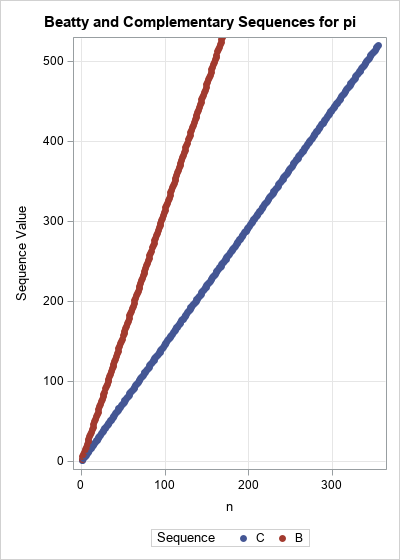The red line has slope π, and the blue line has slope π/(π-1). The domain of these functions contains the integers in [1, 355]. The range of the red line is the Beatty sequence for π; the range of the blue line is the complementary sequence.

Because of the scale, it is difficult to determine how the range of the two linear functions interlace. Let's print out a few values of the interlaced sequences:

proc print data=Beatty(obs=12) noobs; var s Sequence; run;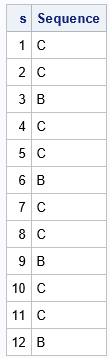The output shows that the first two integers are from the complementary sequence whereas the third is from the Beatty sequence for π. I call this the "two out, one in" pattern. This pattern continues for the first 12 observations. Now, clearly this pattern cannot continue forever, or else 1/3 of the positive integers would be in the Beatty sequence. However, we know that (1/π)th of the integers belong to the Beatty sequence by considering the limit of the ratio n/Bn as n → ∞. Thus, slightly less than 1/3 of the integers are in the Beatty sequence. Let's plot the first 60 positive integers and visualize whether each integer belongs to the Beatty sequence or the complementary sequence, as follows:

data SeqViz; set Beatty(where=(s<=60)); z = 1; /* for plotting the points as a strip plot */ run;   ods graphics / width=640px height=150px; title "Beatty and Complementary Sequences for pi"; title2 "First 60 Integers"; proc sgplot data=SeqViz noautolegend; where s<=60; yaxis display=none; xaxis grid gridattrs=(color=CXF0F0F0) values=( 0 to 21 by 3 25 to 43 by 3 47 to 60 by 3 ) valueshint; scatter x=s y=z / group=Sequence datalabel=Sequence datalabelpos=top datalabelattrs=(size=12) markerattrs=(symbol=SquareFilled size=12); run;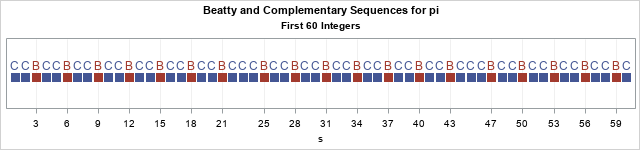The graph shows that every so often there are three (rather than two) consecutive elements of the complementary sequence. This first happens for the integers 22, 23, and 24. The fact that the "extra" element of the complementary sequence appears at 22 is not random. It is related to the fact that 22/7 is a good approximation to π. After that, the pattern returns to "two out, one in" until the next multiple of 22, which is 44, when another triplet of integers belong to the complementary sequence.

The pattern deviates again for other numerators that are associated with the continued fraction expansion of π, such as 355/113. The next graph shows the membership of integers in the range [302, 361]. The pattern of "two out, one in" is broken at 308 = 22*14 and at 330=22*15, but then the pattern deviates again at 355, which is not a multiple of 22.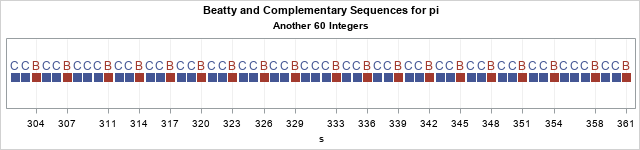### Check that the Beatty and complementary sequences are disjoint

We have asserted that the Beatty and complementary sequences are disjoint and that their union is the set of all positive integers. You can't use numerical computations to prove this fact, but you can verify that it is true for the finite set of numbers that we have generated. The following SAS/IML program checks that the two sequences do not intersect and that their union covers all positive integers (up to 520).

/* Check union and intersection properties of Beatty and complementary sequences */ proc iml; use Beatty; read all var 's' into B where(Sequence='B'); read all var 's' into C where(Sequence='C'); close;   /* is there any intersection? */ intersect = xsect(B, C); if IsEmpty(intersect) then print "B and C do not intersect"; else print "The intersection is:", intersect;   /* use the UNION function */ max = min( max(B), max(C) ); union = union(B, C); union = union[ , 1:max]; /* only test for values in [1, max] */ if all(union = 1:max) then print "All integers are found"; else print "Some integer is missing"; QUIT;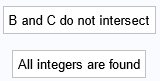The program verifies (for positive integers less than 520) that the Beatty and complementary sequences do not have any intersection. Furthermore, each integer is either an element of the Beatty sequence for π or it is an element of the complementary sequence.

I want to point out a cool SAS/IML syntax: The IF-THEN statement can be used to test whether one vector equals another vector. In the program, the statement all(union = 1:max) returns true is every element of the vector union is equal to the corresponding element of the vector {1,2,3,...,max}.

### Summary

This article describes Beatty's theorem, which is an interesting result in mathematics. Given any irrational number, r > 1, the Beatty sequence for r and the complementary sequence partition the positive integers into two disjoint groups: the Beatty sequence for r and the complementary sequence. The article illustrates the theorem by using r = π and shows some connections with the continued fraction expansion of π.

The post The Beatty sequence for pi appeared first on The DO Loop.For some reason, SAS programmers like to express their love by writing SAS programs. Since Valentine's Day is next week, I thought I would add another SAS graphic to the collection of ways to use SAS to express your love.

Last week, I showed how to use vector operation and a root-finding algorithm to visualize the path of a ball rolling on an elliptical billiards table. Every time the ball hits the boundary of the table, it rebounds and continues rolling in a new direction. At the end of the article, I commented that "the same program can compute the path of a ball on any table" for which certain conditions hold. With Valentine's Day coming soon, I wondered whether I could visualize the dynamics of a ball bouncing across a heart-shaped table. The graph to the right is the result of my efforts.

In the figure, the pink area is the interior of the heart-shaped billiard table. The red lines trace the path of a ball that starts in the middle of the heart with an initial motion at 45 degrees to the horizontal. The figure shows the ball's path for the first 250 bounces.

Although the caption of the figure is "Love Makes My Heart Bounce," the figure might also resonate with people who feel like they bounce around and never find love. It might appeal to those who have recently ended a relationship and feel like they are on the rebound. And during the global pandemic, maybe you have stayed close to home, never venturing very far from those you love? This heart is for you, too!

### Details of the computation

Here are a few details about the computation:

• The shape of the heart is defined by $H(x,y)=0$, where $H(x,y) = (x^2 + y^2 - 1)^3 - x^2 y^3$.
• The gradient of H vanishes at the four points on the curve, namely (0, ±1) and (±1, 0). If the virtual ball "hits" the table's virtual boundary at those locations, the ball will "stick" to the boundary because the program uses the gradient of the function to compute the normal vector to the curve. The program could be modified to compute the normal vector at (±1, 0). However, the curve has a cusp singularity at (0, ±1), so it is impossible to define a normal vector at those points. On a real billiard table, you would want to round off the singularity at those points.
• Because the heart shape is not convex, I had to modify the routine that finds the intersection of a ray and the curve H=0. The previous article on this topic assumes that a ray intersects the curve at only one point. Along the top of the heart, a ray might intersect the curve at three points. I modified the program to find the nearest intersection point.

You can download the program that I used to make the graph. Feel free to change the colors and caption and spread the love.

The post Billiards on a heart-shaped table appeared first on The DO Loop.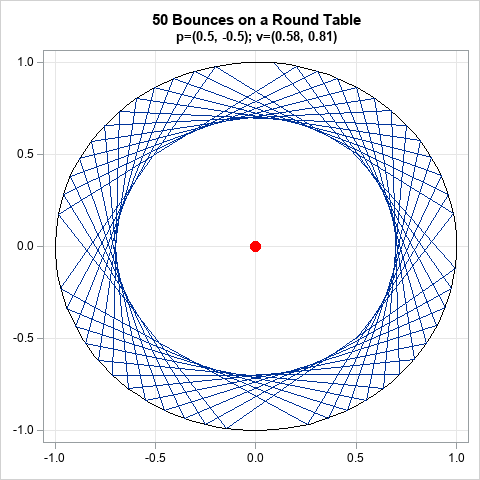I recently showed how to find the intersection between a line and a circle. While working on the problem, I was reminded of a fun mathematical game. Suppose you make a billiard table in the shape of a circle or an ellipse. What is the path for a ball at position p on the table if it rolls in a specified direction, as determined by a vector, v? A possible path is shown in the graph at the right for a circular table. The figure shows the first 50 bounces for a ball that starts at position p=(0.5 -0.5) and travels in the direction of v=(1, 1).

This article shows how to use SAS to compute images like this for circular and elliptical tables.

### Vectors and geometry

One of the advantages of the SAS/IML matrix language is its natural syntax: You can often translate an algorithm from its mathematical formulation to a computer program by using only a few statements. This section presents the mathematical formulation for finding the trajectories of a ball on a circular or elliptical billiard table, then shows how to translate the math into SAS/IML statements.

The fundamental mathematical assumption is that all collisions are perfectly elastic and that the angle of incidence equals the angle of reflection. For a curved surface, the angles are measured by using the normal and tangent vectors to the curve. The geometry is shown in the following figure.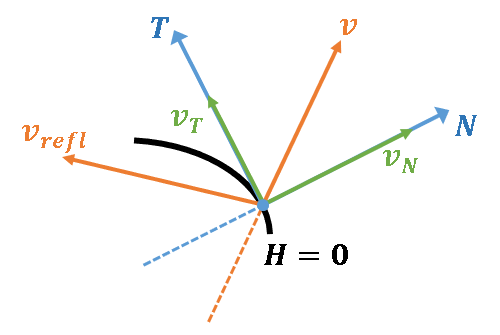A ball moves toward the boundary of the table with velocity vector v. The boundary is represented as the level set H = 0 for some bivariate function, H(x). For example, a circular table uses H(x,y) = x2 + y2 - 1. The previous article showed how to find the point at which the ball hits the curve.

You can compute the unit normal vector, N, at that point by using the gradient of H. Project the velocity vector onto the unit normal to obtain vN. The linear space orthogonal to N is the tangent space. The vector vT = v - vN is the projection of v onto the tangent space. (Note that v = vT + vN.) The reflection of v is defined to be the vector that has the same tangent components but the opposite normal components, which is vrefl = vT - vN. A little algebra shows that vrefl = v - 2vN, so, in practice, the projection onto the tangent space is not needed.

The following SAS/IML program implements functions that compute these vectors. To test the functions, assume a ball is initially at the point p=(0.5, -0.5) and moves with unit velocity v=(1, 1)/sqrt(2), which is 45 degrees from the horizontal.

proc iml; /* Define the multivariate function H and its gradient. Example: H(x)=0 defines a circle and grad(H) is the gradient */ start H(x); return( x[ ,1]##2 + x[ ,2]##2 - 1 ); /* = x##2 + y##2 - 1 */ finish; start gradH(x); return( 2*x ); /* { dH/dx dH/dy } */ finish;   /* given x such that H(x)=0 and a unit vector, v, find the normal and tangent components of v such that v = v_N + v_T, where v_N is normal to H=0 and v_T is tangent to H=0 */ /* project v onto the normal space at x */ start NormalH(x, v); grad = gradH(x); /* if H(x)=0, grad(H) is normal to H=0 at x */ N = grad / norm(grad);/* unit normal vector to curve at x */ v_N = (v*N) * N; /* projection of v onto normal vetor */ return( v_N ); finish; /* project v onto the tangent space at x */ start TangentH(x, v); v_N = NormalH(x, v); v_T = v - v_N; return( v_T ); finish; /* reflect v across tangent space at x */ start Reflect(x, v); v_N = NormalH(x, v); v_refl = v - 2*v_N; return( v_refl ); finish;   /* Test the definitions for the following points and direction vector */ p = {0.5 -0.5}; /* initial position of ball */ v = {1 1} / sqrt(2); /* unit velocity vector: sqrt(2) = 0.7071068 */ q = {1 0}; /* ball hits circle at this point */ N = NormalH(q, v); /* normal component to curve at q */ T = TangentH(q, v); /* tangential component to curve at q */ v_refl = Reflect(q, v); /* reflection of v */ print v, N, T, v_refl;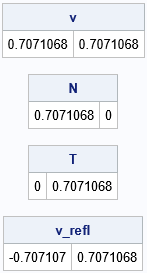From the initial position and velocity, it is clear that the ball will hit the circle at q=(1,0) and reflect off at 135 degrees, which is the direction vector vrefl=(-1, 1)/sqrt(2). For this geometry, the unit normal vector at q is N=(1,0) and the unit tangent vector is T=(0,1), so it is easy to mentally verify that the program is giving the correct values for the vectors in this problem.

### Bouncing around a billiard table

Let's play a game of billiards on a table that has a nonstandard shape. To begin the game, you must specify the initial position (p) and velocity (v) of the ball, as well as the shape of the table (a function H). The algorithm is simple:

1. Given p and v, find the point q where the ball hits the boundary of the table. This step is described in a previous article..
2. Reflect v across the normal vector at q to obtain a new direction, vrefl.
3. Repeat this process some number of times. Optionally, plot the path to visualize how the ball bounces across the table.

The following SAS/IML program implements this algorithm by using the F_Line and FindIntersection functions from the previous article:

/* To find the intersection of H=0 with the line through G_p in the direction of G_v, see https://blogs.sas.com/content/iml/2022/02/02/line-search-sas.html */ /* Restriction of H to the line through G_p in the direction of G_v */ start F_Line(t) global(G_p, G_v); return( H( G_p + t*G_v ) ); finish; /* return the intersection of H=0 and the line */ start FindIntersection(p, v, step=20) global(G_p, G_v); G_p = p; G_v = v; t = froot("F_Line", 1E-3 || step); /* find F(t) = 0 for t in [1E-3, step] */ q = p + t*v; /* H(q) = 0 */ return( q ); finish; /* given a point, p, and a direction vector, v, find the point q on the line through p in the direction of v such that H(q)=0. Reflect the velocity vector to get a new direction vector, v_refl */ start HitTable(q, v_refl, p, v); q = FindIntersection(p, v); v_refl = Reflect(q, v); finish;   /* Example: A periodic path with four segments */ p = {0.5 -0.5}; /* point p */ v = {1 1}/sqrt(2); /* direction vector v */   NSeg = 6; /* follow for this many bounces */ x = j(NSeg, ncol(p)); x[1,] = p; do i = 2 to NSeg; run HitTable( q, v, x[i-1,], v ); /* overwrite v on each iteration */ x[i,] = q; end; print x[L="Positions"];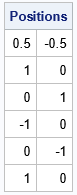These initial conditions are highly symmetric and therefore easy to visualize. From the initial position and velocity, the ball hits the boundary of the circular table at (1,0), (0,1), (-1,0), and (0,-1) before returning again to (1,0). The ball will follow this periodic trajectory forever. It will always hit the same four points on the boundary of the circle.

For a general initial condition, you will want to draw a graph that visualizes the orbit of the ball as it bounces across the table. You can download the SAS program that computes the graphs in this article. For ease of use, I encapsulated the computations and visualization into a module called Billiards. You can call the Billiards subroutine with an initial condition and the number of bounces and the module will return a graph of the trajectory of the ball. For example, the following statements generate the first 50 bounces of a ball on a circular table:

/* Example 2. Either periodic orbit or bounces inside an annulus */ p = {0.5 -0.5}; /* point p */ v = {1 1.4}; /* vector v */ v = v / norm(v); /* standardize to unit vector */ print v;   title "50 Bounces on a Round Table"; title2 "p=(0.5, -0.5); v=(0.58, 0.81)"; run Billiards(p, v, 50); /* NSeg=50 bounces */

The graph is shown at the top of this article. For this example, the ball bounces around the table inside an annular region. For a circular table, you can prove mathematically that every trajectory is either a periodic orbit or is dense in an annular region.

### Billiards on an elliptical table

If you study the previous program, you will see that the program does not use any special knowledge of the shape of the table. Given any smooth functions H and grad(H), the program should be able to handle tables of other shapes. That is the advantage of writing a program that uses vectors in a coordinate-free manner.

Let's change the functions for H and grad(H) to use an elliptical table with parameters a and b. That is, the shape of the table is determined by H(x)=0, where $H(\mathbf{x}) = x_1^2/a^2 + x_2^2/b^2 - 1$ is the standard form of an ellipse. For the next example, let's use a=sqrt(5) and b=2.

/* define the elliptical region and gradient vector */ start H(x); a = sqrt(5); b = 2; return( (x[ ,1]/a)##2 + (x[ ,2]/b)##2 - 1 ); /* ellipse(a,b) */ finish; start gradH(x); a = sqrt(5); b = 2; return( 2*x[ ,1]/a##2 || 2*x[ ,2]/b##2 ); finish;

An ellipse is determined by its two foci, which are located at (+/-sqrt(a2 - b2), 0) when a > b. For our example, the foci are at (-1, 0) and (1, 0).

You can show that the path of a ball on an elliptical table is one of three shapes:

• If the ball does not pass between the foci, its path is inside an annular region.
• If the ball passes between the foci, its path is inside a hyperbolic region.
• If the ball passes over one focal point, it also passes over the second focal point. The path converges to the major axis that contains the foci.

The following examples show each case:

/* If the ball does not pass between the foci, its path is inside an annular region. */ p = {1.5 0}; /* point p */ v = {0 1}; /* vector v */ title "250 Bounces on an Elliptical Table"; title2 "Ball Does Not Pass Between Foci"; run Billiards(p, v, 250);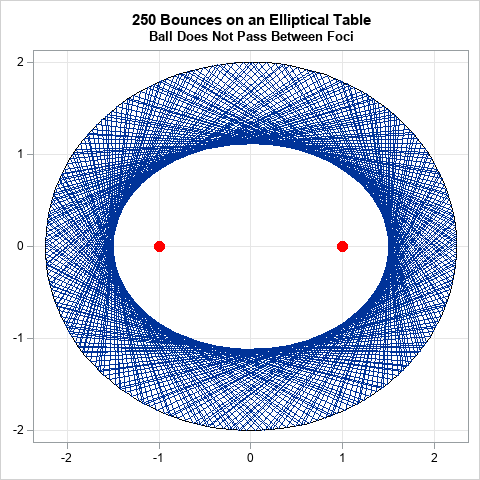/* If the ball passes between the foci, its path is inside a hyperbolic region. */ p = {0 0}; /* point p */ v = {1 1}/sqrt(2); /* vector v */ title "250 Bounces on an Elliptical Table"; title2 "Ball Passes Between Foci"; run Billiards(p, v, 250);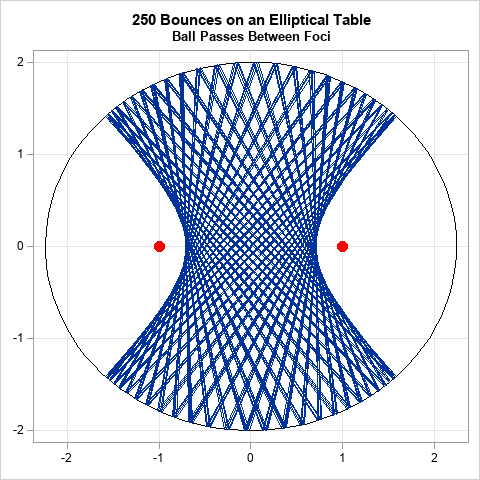/* If the ball passes over one focal point, it also passes over the second focal point. The path converges to the major axis that contains the foci. */ p = {1 -1}; /* point p */ v = {0 1}; /* vector v */ title "10 Bounces on an Elliptical Table"; title2 "Ball Passes Through a Focal Point"; run Billiards(p, v, 10);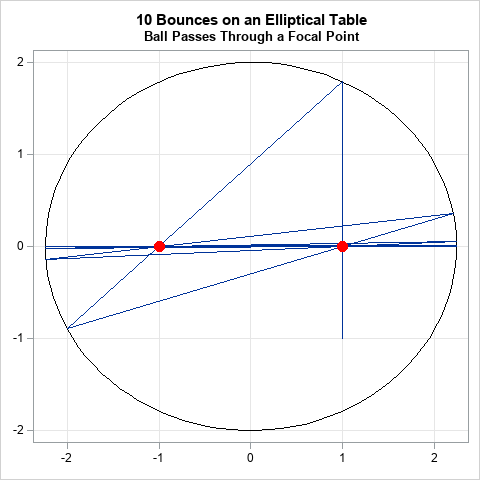### Summary

In summary, you can use analytical geometry to figure out the trajectory of a ball on nonstandard billiard table that has a curved boundary. By using vectors and a root-finding algorithm in the SAS/IML language, you can write a program that displays the path of a ball on a circular table. The advantage of a coordinate-free vector formulation is that the same program can compute the path of a ball on a table that has a more complicated boundary, such as an ellipse. In fact, the same program can compute the path of a ball on any table for which H=0 defines a smooth convex curve on which the gradient of H does not vanish.

Incidentally, people have built elliptical billiard tables and have put a hole at one of the foci. To sink a ball, you can shoot it at the hole or you can shoot it at the other focal point. Either way, the ball rolls into the hole.

The post Billiards on an elliptical table appeared first on The DO Loop.

Suppose you are creating a craft project for the Christmas holidays, and you want to choose a palette of Christmas colors to give it a cheery holiday appearance. You could use one of the many online collections of color palettes to choose a Christmas-themed palette. However, I didn't want to merely take a palette that someone else designed. As a statistician, I wanted to create my own Christmas palette by using some sort of mathematical technique that creates a "statistically perfect" set of Christmas colors from other palettes!

Artists use their talents to create aesthetically pleasing and harmonious palettes of colors. But why should artists have all the fun? In this article, I show how a statistician might create a palette of Christmas colors! Starting from existing palettes of colors, the technique uses principal component analysis to select a linear subspace of colors. From within that subspace, you can create your own palette of colors. An example is the green-gold-red palette that is shown below. It was made by math, but I think it is pretty!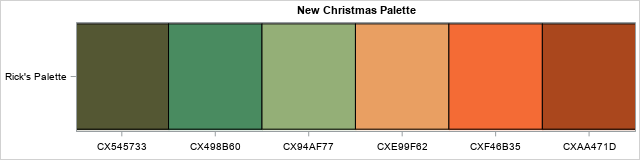The same technique could also be used to create autumn palettes, spring palettes, and so forth, but don't take this too seriously: this article is intended to be geeky fun for the holidays. I'll let others debate whether this mathematical technique is useful for creating art.

### Outline of the method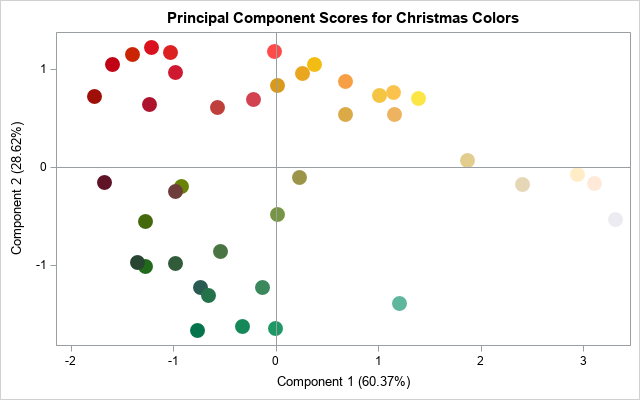Here is the general idea behind creating a statistical palette of colors:

1. Collect 6-10 existing palettes that you like. This process should produce 40-60 colors that are related to your theme (such as "Christmas"). I have written about how to read and visualize palettes in SAS.
2. Each color can be represented a triplet of values in RGB space. You can use principal component (PC) analysis to project the colors into a plane that captures most of the variation in the colors. The graph at the right shows the principal component scores for 41 colors in seven Christmas palettes.
3. Each palette is represented as a path through this linear subspace. So, you can choose a new sequence of points in this subspace and then REVERSE the projection to obtain a new palette of RGB colors.
4. There are infinitely many paths you could choose, but one choice is to use vertices of a regular polygon to create a "statistically perfect" set of Christmas colors.

### Paths through principal component space

For each palette, if you connect the sequence of colors in the palette, you obtain a path through the plane of the principal component scores. If you try to display the paths for all seven palettes, you will get a jumbled mess of lines that crisscross the graph. To make it easier to visualize the paths for each palette, the following graph limits the display to two palettes. The "Jingle Bell" palette has six colors. It starts with two green colors, moves to golden colors, and ends with two red colors. The "Unwrapping My Gifts" palette has five colors. It starts with two green colors, moves to a silver color, and ends with a red and dark burgundy color. The other palettes trace similar paths through the plane of scores.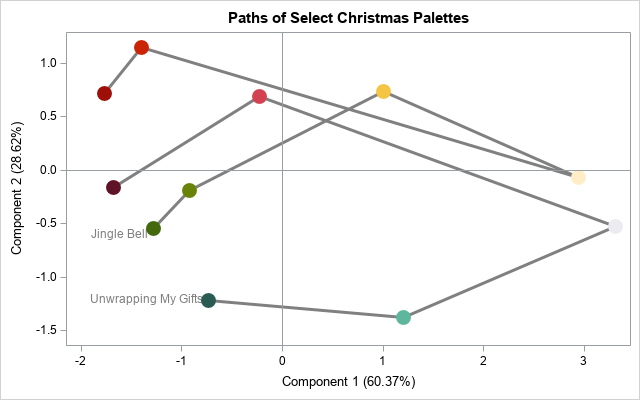If each palette corresponds to a path, then each path corresponds to a palette. In other words, if you choose a sequence five or six coordinates in the space of principal component scores, the path defines a new Christmas palette.

The ancient Greeks thought that a circle was the most perfect shape. Regular polygons, which approximate the circle, are also seen as perfect in their symmetry and beauty. Therefore, it makes sense to use a regular hexagon to create a new Christmas palette. The following graph demonstrates how you can choose a new Christmas palette. The circle represents a path through the space of colors. The gray stars represent six points on the circle, which are also vertices on a regular hexagon. I will define a new palette by using math to figure out what colors to associate with the coordinates of the six stars. I've ordered the six points starting from the point that I know will be green (because it is in the lower-left corner) and ending with the point that I know will be red (because it is in the upper-left corner).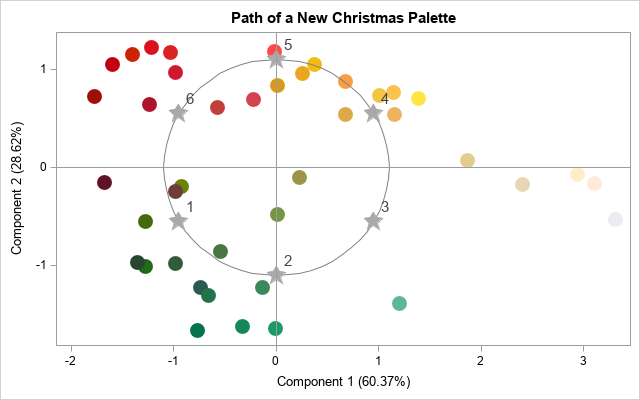### Inverting the principal component transformation

Until now, we've only considered the projection of the RGB colors onto the plane of the first two PCs. Of course, this projection is not invertible: There are many colors that share the same two PC scores. However, in general, the transformation from RGB space to the space of all principal component scores is invertible. You can represent the transformation as a matrix by using the spectral decomposition of the correlation matrix of the colors. To embed the plane of the first two PCs, you need to set a value for the coordinate of the third PC. The obvious statistical choice is to use PC3=0, which represents the average score in the third PC. In other words, you can associate the first two PC scores with the plane that has ordered triplets (PC1, PC2, 0).

With that choice, it is straightforward to apply the inverse PC transformation. The inverse transformation maps PC scores into colors in RGB space. You can use the SAS IML language to carry out this transformation. If you visualize a dense set of points on the circle, you get the following spectrum of colors: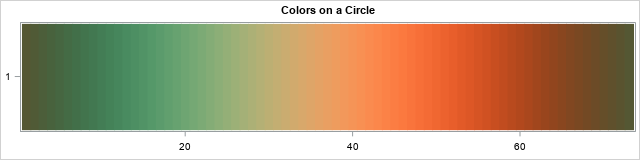Any smaller palette will contain a subset of these colors. For example, if you choose six points on the circle that are vertices of a regular histogram, you get the palette that is shown at the top of this article. The new palette is based on the original seven palettes but is distinct from them. The new palette incorporates the properties of the original palettes but is defined by defining a new path through the PC scores.

### Summary

In summary, this article shows how to create a new palette of colors that is based on statistical properties of a set of colors. You can perform a principal component analysis in RGB space to obtain a plane of PC scores. Every path through that plane corresponds to a new palette of colors. For my palette, I chose a sequence of vertices on a regular hexagon.

This method is not guaranteed to give an aesthetically pleasing palette of colors, but I think it does in this example. And if you don't like my palette, you can choose any other path through the set of PC scores to create a new palette! If you would like to experiment with this technique, you can download the SAS program that I used to generate the figures in this article.

The post A statistical palette of Christmas colors appeared first on The DO Loop.

The number of possible bootstrap samples for a sample of size N is big. Really big.

Recall that the bootstrap method is a powerful way to analyze the variation in a statistic. To implement the standard bootstrap method, you generate B random bootstrap samples. A bootstrap sample is a sample with replacement from the data. The phrase "with replacement" is important. In fact, a bootstrap sample is sometimes called a "resample" because it is generated by sampling the data with REplacement.

This article compares the number of samples that you can draw "with replacement" to the number of samples that you can draw "without replacement." It demonstrates, for very small samples, how to generate the complete set of bootstrap samples. It also explains why generating a complete set is impractical for even moderate-sized samples.

### The number of samples with and without replacement

For a sample of size N, there are N! samples if you sample WITHOUT replacement (WOR). The quantity N! grows very quickly as a function of N, so the number of permutations is big for even moderate values of N. In fact, I have shown that if N≥171, then you cannot represent N! by using a double-precision floating-point value because 171! is greater than MACBIG (=1.79769e+308), which is the largest value that can be represented in eight bytes.

However, if you sample WITH replacement (WR), then the number of possible samples is NN, which grows even faster than the factorial function! If the number of permutations is "big," then the number of WR samples is "really big"!

For comparison, what do you think is the smallest value of N such that NN exceeds the value of MACBIG? The answer is N=144, which is considerably smaller than 171. The computation is shown at the end of this article.

Another way to compare the relative sizes of N! and NN is to print a few values of both functions for small values of N. The following table shows the values for N≤10:

proc iml; N = 1:10; nPerm = fact(N); /* N! */ nResamp = N##N; /* N^N */ both = nPerm // nResamp; print both[r={"Permutations" "Samples (WR)"} c=N format=comma15. L=""];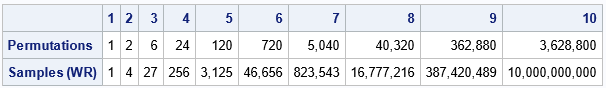Clearly, the number of bootstrap samples (samples WR) grows very big very fast. This is why the general bootstrap method uses random bootstrap samples rather than attempting some sort of "exact" computation that uses all possible bootstrap samples.

### An exact bootstrap computation that uses all possible samples

If you have a VERY small data set, you could, in fact, perform an exact bootstrap computation. For the exact computation, you would generate all possible bootstrap samples, compute the distribution on each sample, and thereby obtain the exact bootstrap distribution of the statistic.

Let's carry out this scheme for a data set that has N=7 observations. From the table, we know that there are exactly 77 = 823,543 samples with replacement.

For small N, it's not hard to construct the complete set of bootstrap samples: just form the Cartesian product of the set with itself N times. In the SAS/IML language, you can use the ExpandGrid function to define the Cartesian product. If the data has 7 values, the Cartesian product will be a matrix that has 823,543 rows and 7 columns.

The following SAS/IML program performs a complete bootstrap analysis of the sample mean. The sample, S, has 7 observations. The minimum value is -1; the maximum value is 4. The sample mean is approximately 0.51. What is the bootstrap distribution of the sample mean? You can form the set of all possible subsamples of size 7, where the elements are resampled from S. You can then compute the mean of every resample and plot the distribution of the means:

proc iml; S = {-1 -0.1 -0.6 0 0.5 0.8 4}; /* 1. compute original statistic */ m = S[:]; /* = mean(S) */ print m;   /* 2. Generate ALL resamples! */ /* Cartesian product of the elements in S is S x S x ... x S \------v------/ N times */ z = ExpandGrid(S, S, S, S, S, S, S);   /* 3. Compute statistic on each resample */ means = z[,:];   /* 4. Analyze the bootstrap distribution */ title "Complete Bootstrap Distribution of the Mean"; title2 "N = 7"; call histogram(means) rebin={-1 0.1} xvalues=-1:4 other="refline 0.51/axis=x lineattrs=(color=red);";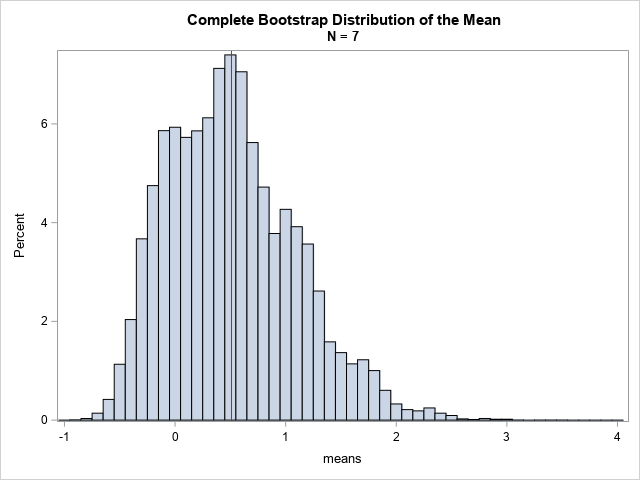The graph shows the mean for every possible resample of size 7. The mean of the original sample is shown as a red vertical line. Here are some of the resamples in the complete set of bootstrap samples:

• The resamples where a datum is repeated seven times. For example, one resample is {-1, -1, -1, -1, -1, -1, -1}, which has a mean of -1. Another resample is {4, 4, 4, 4, 4, 4, 4}, which has a mean of 4. These two resamples define the minimum and maximum values, respectively, of the bootstrap distribution. There are only seven of these resamples.
• The resamples where a datum is repeated six times. For example, one of the resamples is {-1, -1, -1, -1, -1, -1, 4}. Another is {-1, -1, 0, -1, -1, -1, -1}. A third is {4, 4, 4, 4, 4, 4, 4}, which has a mean of 4. These two resamples define the minimum and maximum values. Another resample is {4, 4, 4, 4, 0.5, 4, 4}. There are 73 resamples of this type.
• The resamples where each datum is present exactly one time. These sets are all permutations of the sample itself, {-1 -0.1 -0.6 0 0.5 0.8 4}. There are 7! = 5,040 resamples of this type. Each of these permutations has the same mean, which is 0.51. This helps to explain why there is a visible peak in the distribution at the value of the sample mean.

The bootstrap distribution does not make any assumptions about the distribution of the data, nor does it use any asymptotic (large sample) assumptions. It uses only the observed data values.

### Comparison with the usual bootstrap method

For larger samples, it is impractical to consider ALL possible samples of size N. Therefore, the usual bootstrap method generates B random samples (with replacement) for a large value of B. The resulting distribution is an approximate bootstrap distribution. The following SAS/IML statements perform the usual bootstrap analysis for B=10,000.

/* Generate B random resamples. Compute and analyze bootstrap distribution */ call randseed(123); w = sample(S, {7, 10000}); /* 10,000 samples of size 7 (with replacement) */ means = w[,:]; title "Bootstrap Distribution of the Mean"; title2 "B = 10,000; N = 7"; call histogram(means) rebin={-1 0.1} xvalues=-1:4 other="refline 0.51/axis=x lineattrs=(color=red);";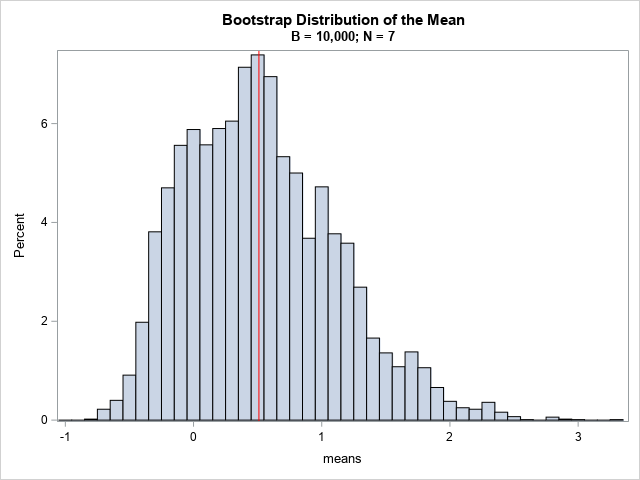The resulting bootstrap distribution is similar in shape to the complete distribution, but only uses 10,0000 random samples instead of all possible samples. You can see that the properties of the second distribution (such as quantiles) will be similar to the quantiles of the complete distribution, even though the second distribution is based on many fewer bootstrap samples.

### Summary

For a sample of size N, there are NN possible resamples of size N when you sample with replacement. The bootstrap distribution is based on a random sample of these resamples. When N is small, you can compute the exact bootstrap distribution by forming the Cartesian product of the sample with itself N times. This article computes the exact bootstrap distribution for the mean of seven observations and compares the exact distribution to an approximate distribution that is based on B random resamples. When B is large, the approximate distribution looks similar to the complete distribution.

For more about the bootstrap method and how to perform bootstrap analyses in SAS, see "The essential guide to bootstrapping in SAS."

### Appendix: Solving the equation NN = y

This article asked, "what is the smallest value of N such that NN exceeds the value of MACBIG?" The claim is that N=144, but how can you prove it?

For any value of y > 1, you can use the Lambert W function to find the value of N that satisfies the equation NNy. Here's how to solve the equation:

1. Take the natural log of both sides to get N*log(N) ≤ log(y).
2. Define w = log(N) and x = log(y). Then the equation becomes w exp(w) ≤ x. The solution to this equation is found by using the Lambert W function: w = W(x).
3. The solution to the original equation is N = exp(w). Since N is supposed to be an integer, round up or down, according to the application.

The following SAS/IML program solves for the smallest value of N such that NN exceeds the value of MACBIG in double-precision.

proc iml; x = constant('LogBig'); /* x = log(MACBIG) */ w = LambertW(x); /* solve for w such that w*exp(w) = x */ N1 = exp(w); /* because N = log(w) */ N = ceil(N1); /* round up so that N^N exceeds MACBIG */ print N1 N; quit;So, when N=144, NN is larger than the maximum representable double-precision value (MACBIG).

The post On the number of bootstrap samples appeared first on The DO Loop.Art evokes an emotional response in the viewer, but sometimes art also evokes a cerebral response. When I see patterns and symmetries in art, I think about a related mathematical object or process. Recently, a Twitter user tweeted about a painting called "Phantom’s Shadow, 2018" by the Nigerian-born artist, Odili Donald Odita. A modified version of the artwork is shown to the right.

The artwork is beautiful, but it also contains a lot of math. The image shows 64 rotations, reflections, and translations of a polygon in four colors. As I will soon explain, the image can also be viewed as 4 x 4 grid where each cell contains a four-bladed pinwheel. The grid displays rotations and reflections of a pinwheel shape.

When I saw this artwork, it inspired me to look closely at its mathematical structure and to create my own mathematical version of the artwork in SAS. This article shows how to use rotations to create a pinwheel from a polygon. A second article discusses how to use rotations and reflections to create a mathematical interpretation of Odita's painting.

### Create a polygon

Look closely at the upper left corner of "Phantom's Shadow." You will see the following pinwheel-shaped figure:If the center of the pinwheel is the origin, then this pinwheel is based on a sequence of 90-degree rotations of the teal-colored polygon about the origin. Each rotation is a different color (teal, orange, blue, and salmon) on a gray background.

You can assign coordinates to the vertices of the teal polygon. If three vertices are on the corners of the unit square, the fourth vertex appears to be at (2/3, 1/3). You can put the vertices into a SAS data set and graph the polygon by using the POLYGON statement in PROC SGPLOT:

%let alpha = 0.333; /* Odita's work uses a vertex as (1-alpha, alpha) */ data Poly1; ID = 1; input x y @@; if x=. then do; /* just for fun, support other locations of vertex */ x = 1 - &alpha; y = &alpha; end; datalines; 0 0 . . 1 1 0 1 0 0 ;   %let blue = CX0f5098; %let orange = CXeba411; %let teal = CX288c95; %let salmon = CXd5856e; %let gray = CX929386;   ods graphics / width=480px height=480px; title "Base Polygon"; proc sgplot data=Poly1 aspect=1; styleattrs wallcolor=&gray; polygon x=x y=y ID=ID / fill fillattrs=(color=&teal) outline; xaxis offsetmin=0.005 offsetmax=0; yaxis offsetmin=0 offsetmax=0.005; run;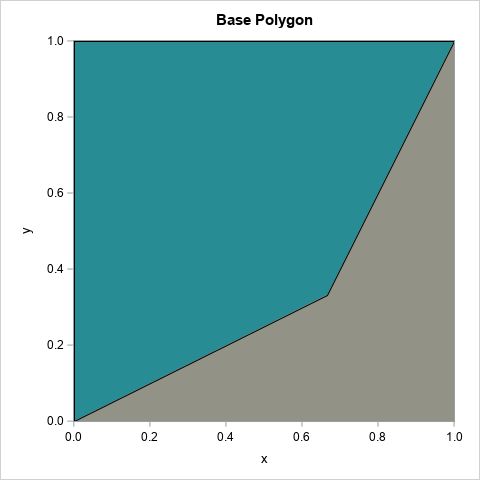The complete artwork repeats this polygon 64 times in a grid by using different colors and different orientations. But the colors and orientations are not random—although that would also look cool! Instead, there is an additional structure. The polygon is part of a pinwheel, and the pinwheel shape is rotated and reflected 16 times in a 4 x 4 grid. The next section shows how to create the pinwheel.

### Create a pinwheel

It is possible to perform 2-D rotations and reflections in the DATA step, but the rotations and reflections of a planar figure are most simply expressed in terms of 2 x 2 orthogonal matrices.

To form the pinwheel from the basic polygon, you can use a group of four matrices. All rotations are in the counter-clockwise direction about the origin:

• R0 is the identity matrix
• R1 is the matrix that rotates a point 90 degrees (about the origin)
• R2 is the matrix that rotates a point 180 degrees
• R3 is the matrix that rotates a point 270 degrees

You do not need advanced mathematics to understand rotations by 90 degrees. However, these four rotation matrices are an example of an algebraic structure called a finite group. These matrices form the cyclic group of order 4 (C4), where the group operation is matrix multiplication.

Regardless of their name, you can use these matrices to transform the polygon into a pinwheel. The following SAS/IML program reads in the base polygon and transforms it according to each of the four matrices. You can then plot the four images, each in a different color.

/* rotate polygon about the origin to form a pinwheel */ proc iml; /* actions of the C4 cyclic group: rotations by 90 degrees */ start C4Action(v, act); if act=0 then M = { 1 0, 0 1}; else if act=1 then M = { 0 -1, 1 0}; else if act=2 then M = {-1 0, 0 -1}; else if act=3 then M = { 0 1, -1 0}; return( v*M ); /* = (M*z) */ finish;   use Poly1; read all var {x y} into P; close; /* read the polygon */   /* write the pinwheel */ Q = {. . .}; create Shape from Q[c={'ID' 'x' 'y'}]; do i = 0 to 3; R = C4Action(P, i); /* rotated image of polygon */ Q = j(nrow(R), 1, i) || R; /* save the image to a data set */ append from Q; end; close; QUIT;   title "Pinwheel Figure"; title2 "Colors=(Teal, Orange, Blue, Salmon)"; proc sgplot data=Shape aspect=1; styleattrs wallcolor=&gray datacolors=(&teal &orange &blue &salmon); polygon x=x y=y ID=ID / group=ID fill; xaxis offsetmin=0 offsetmax=0; yaxis offsetmin=0 offsetmax=0; run;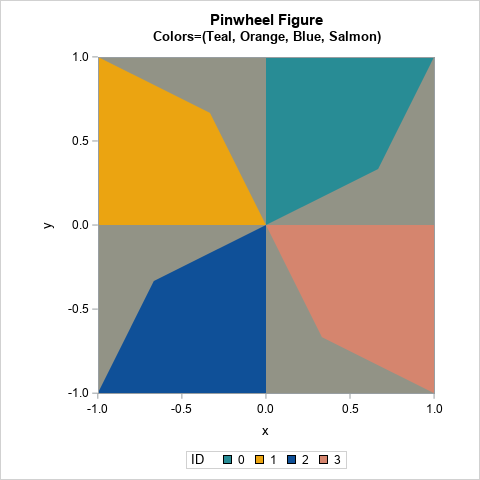The resulting image is the four-blade pinwheel, which is the basis of Odita's artwork.

### A grid of pinwheels

The rest of "Phantom’s Shadow, 2018" can be described in terms of rotations and reflections of the pinwheel shape...with a twist! The next article introduces the dihedral group of order 4 (D4). This is a finite group of order eight and is the symmetry group of the square. It turns out that you can use the elements of D4 to generate the 16 pinwheels in Odita's artwork. You can then use PROC SGPANEL in SAS to generate a graph that looks like "Phantom's Shadow," or you can create your own Odita-inspired mathematical artwork! Stay tuned!

The post The art of rotations and reflections appeared first on The DO Loop.Odani's truism is a mathematical result that says that if you want to compare the fractions a/b and c/d, it often is sufficient to compare the sums (a+d) and (b+c) rather than the products a*d and b*c. (All of the integers a, b, c, and d are positive.) If you compare the products, you will know the correct answer 100% of the time because a/b < c/d if and only if a*d < b*c. However, K. Odani proved (1998) that the expression (a+d) < (b+c) is true 91% of the time when the integers a, b, c, and d are chosen uniformly at random in the interval [1,N], where N is a very large integer. A previous article shows a Monte Carlo simulation that demonstrates Odani's truism.

As discussed at the end of the previous article, the 91% probability includes many fractions that are easy to compare in your head, such as 6/29 versus 35/18, and 1/6 versus 5/8. Odani's result also includes unreduced fractions such as 2/4 and 3/6. What is the probability that comparing the sums gives the correct answer if you consider only reduced fractions a/b and c/d in the interval [0,1] where the fractions are close to each other? That is the subject of this article. Briefly, this article examines whether Odani's truism has any value in practice.

Given a pair of fractions a/b < c/d, we say that Odani's truism is "true" (or valid or correct) if (a+d) < (b+c). Otherwise, we say that the truism is "false."

### The Farey sequence

I will use the Farey sequence in this investigation. The Farey sequence of order n is the sequence of all reduced fractions on [0,1] whose denominators do not exceed n, listed in increasing order. In practice, we do not usually need to compare fractions with huge denominators. Most of the time, we encounter fractions whose denominators are less than 100, which corresponds to the Farey sequence of order 100.

Consider the Farey sequence of order n for a small value of n, such as n=100. The sequence is in increasing order, so you can compare each term of the sequence with the next k terms and compute how often Odani's truism is correct. When k is small compared to n, this method estimates how often Odani's truism is valid for fractions in [0,1] that are near each other.

### First attempt: Fractions that are adjacent in the Farey seqence

Before looking a the general case of k > 0, let's look at the special case where k=1. For each fraction in the Farey sequence, a/d, let c/d be the next fraction in the Farey sequence. Because the Farey sequence is in order, we know that a/b < c/d. Compute the Odani sum (a+d)-(b+c). If the sum is negative, then Odani's truism is correct for that a/b and c/d. Otherwise, the truism is false.

The following SAS/IML function computes the Farey sequence for any order n:

proc iml; /* Generate Farey sequence of order n: all reduced fractions on [0,1] whose denominators do not exceed n, listed in increasing order https://blogs.sas.com/content/iml/2021/03/17/farey-sequence.html */ start Farey(n); /* upperbound on sequence length is n*(n+3)/2. See https://oeis.org/A005728 */ F = j(2, max(2,n*(n+3)/2), .); F[,1] = {0, 1}; F[,2] = 1 // n; order = 2; a=0; b=1; c=1; d=n; /* fractions are a/b and c/d */ do while (d > 1); order = order+1; k = floor((n + b)/d); /* integer division */ a_prev = a; a = c; b_prev = b; b = d; c = k*c - a_prev; d = k*d - b_prev; F[,order] = c // d; end; /* remove any unassigned elements */ keepidx = 1:order; return( F[ ,keepidx] ); finish;

You can call the function generate the Farey sequence for n=100. Let's print out a few fractions in the sequence along with an indicator variable that shows whether their Odani sums are negative:

S = Farey(100); /* generaste Farey sequence of order n=100 */   idx = 401:415; /* look at a few terms in the Farey sequence */ s0 = S[,idx]; /* get the corresponding fractions */ /* evaluate the Odani sum on adjacent pairs */ i = 1:ncol(idx)-1; a = S0[1,i]; b = S0[2,i]; /* first row of S contains numerators; second row contains denominators */ c = S0[1,i+1]; d = S0[2,i+1]; OdaniSum = (a+d)-(b+c); /* when sum is negative, the truism is true (predicts that a/b < c/d) */ Truism = (OdaniSum < 0); /* In SAS/IML 15.1, you can use numeric value for the COLNAME= option. See https://blogs.sas.com/content/iml/2019/07/31/numeric-column-header-print.html */ print (s0//(Truism||.))[L="Some Fractions in Farey(100)" c=idx r={"Numer" "Denom" "Truism"}];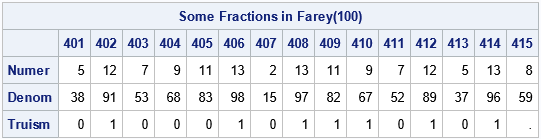The Farey sequence of order 100 has 3,045 elements. The table shows the values for the elements near the fraction 2/15, which is the 407th element in the sequences. The program compares the i_th fraction to the (i+1)st fraction and computes whether (a+d) < (b+c) for the adjacent pairs. For the 14 comparisons in this example, the Odani truism was true for seven and false for the other seven.

A 50% success rate is not very good! Maybe fractions near 2/15 are "unusual" in some way? Let's compare all adjacent pairs of fractions in the Farey(100) sequence and compute the proportion that satisfies Odani's truism. We can also plot the truth or falsity of Odani's truism for each fraction in the Farey sequence:

/* compare each fraction to the one that follows it */ i = 1:ncol(S)-1; /* the indices of a/b; i+1 contains the indices of c/d */ a = S[1,i]; b = S[2,i]; c = S[1,i+1]; d = S[2,i+1]; Truism = ((a+d)-(b+c) < 0);   /* plot the result (T or F) versus the fraction */ title "Odani's Truism for Consecutive Fractions in Farey(100)"; x = S[1,i] / S[2,i]; TorF = choose(Truism=1, "True ", "False"); call scatter(x, TorF) grid={x y} option="transparency=0.95" xvalues=do(0,1,0.1);   prob = mean(Truism); print prob;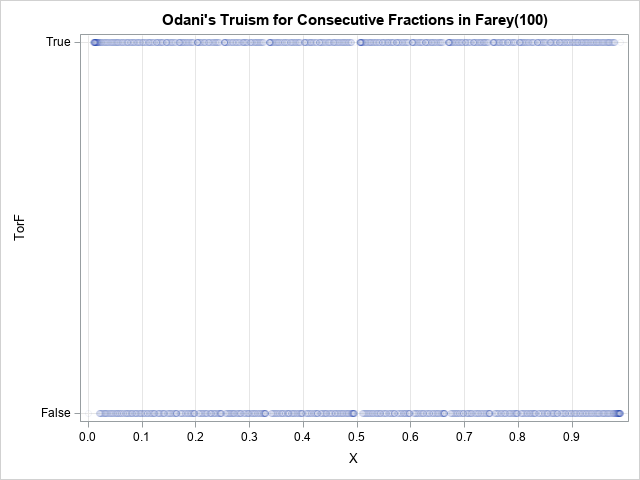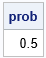When applied to adjacent fractions in the Farey sequence, Odani's truism is true only 50% of the time. The scatter plot shows the truth or falsehood of Odani's truism for each fraction in the Farey sequence. The results do not seem to depend on the location of the fractions within the interval [0,1].

Notice that this result does not violate Odani's theorem because we are comparing only certain fractions: reduced fractions in [0,1] that are adjacent to each other in the Farey sequence.

### Compare fractions that are k terms away in the Farey seqence

For a Farey sequence that contains L terms, the previous section shows how to compare L-1 pairs of fractions. Each fraction was compared to the next fraction in the Farey sequence. If we perform ALL pairwise comparisons of the terms in the Farey sequence, Odani's truism will be valid for a larger proportion of comparisons. It probably won't hold for 91% of the comparisons (because we are dealing with reduced fractions in [0,1]), but I expect it to hold for more than 50%.

Let L be the length of the Farey sequence. The following function compares each term in the Farey sequence with the next k terms in the sequence, when possible. For the first L-k terms, you can compare each term with the k terms that follow. For the last k terms, you can only compare to the end of the sequence. Thus, there are a total of k*(L-k) + k*(k-1)/2 comparisons. If you count the number of pairs that satisfy Odani's truism and divide by the total number of comparisons, you get the proportion of comparisons for which Odani's sum accurately predicts whether one fraction is smaller than another:

/* S is a Farey sequence. k is the number of subsequent terms that we compare to each fraction. For example: k = 1 will compare adjacent terms k = 2 will compare each fraction with the following two terms, and so on... */ start CompareFract(S, k); Len = ncol(S); count = 0; numCompared = k*(Len-k) + k*(k-1)/2; /* total number of pairwise comparisons */ do i = 1 to Len-1; a = S[1,i]; b = S[2,i]; /* i = the indices of a/b; /* we know that a/b is less than all the fractions that follow */ idx = (i+1):min(i+k, Len); /* the next 'k' fractions or until the end */ c = S[1, idx]; d = S[2, idx]; /* c/d for the next k fractions */ OdaniSum = (a+d) - (b+c); count = count + sum(OdaniSum<0); /* how many fractions satisfy the eqn? */ end; prop = count / numCompared; /* proportion of comparisons that satisfy eqn */ return count || numCompared || prop; finish;

Let's apply the function to the Farey sequence of order 100, which has 3,045 terms. Let's perform a total of 100 comparisons, where k is evenly distributed among the values 1–3045. (The number of comparisons, 100, is unrelated to the order of the Farey sequence.) The following statements call the CompareFracts function for values of k that are approximately 3045/100 units apart. The exact values are k={30, 60, 91, ..., 3014, 3045}.

S = Farey(100); pct = do(0.01, 1.0, 0.01); /* evenly spaced percentages */ k = int( ncol(S)*pct ); /* choose k to be percentage of sequence length */ results = j(ncol(k), 3, .); do i = 1 to ncol(k); results[i,] = CompareFract(S, k[i]); end;   title "Proportion of Odani Comparisons That Are True"; title2 "Farey Sequence of Order 100"; refStmt = "refline 0.917 / axis=y noclip label='11/12';"; call series(pct, results[,3]) grid={x y} other=refStmt label={'Fraction of Sequence Length' 'Proportion'};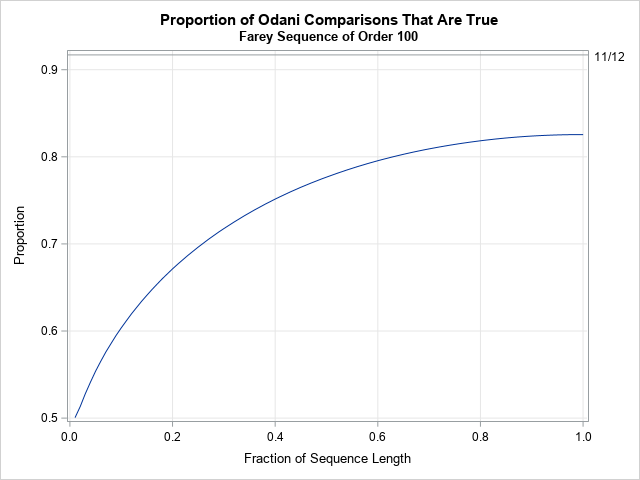The graph shows the percentage of comparisons for which Odani's truism is valid when comparing nearby fractions in the Farey sequence of order 100. The horizontal axis displays the proportion of the sequence that is being compared: 1%, 2%, ..., 100%. The left side of the graph indicates that if you compare each fraction to a small number of fractions that are close to it (such as 1% of the sequence length), Odani's truism is valid only about 50% of the time. If you compare each fraction to more fractions (which are, by definition, farther away from it), then Odani's truism is correct for a larger proportion of comparisons. If you consider all pairwise comparisons of the fractions, you find that about 82.5% of the comparisons satisfy Odani's truism (for the fractions in this Farey sequence).

A similar curve is seen when you consider larger sets of fractions. For example, for the Farey sequence of order 500 (which contains the 76,117 fractions whose denominators are less than or equal to 500), the graph looks similar except it is slightly higher: when you compare all fractions, Odani's truism is valid 83.2% of the time. As you consider larger Farey sequences, the proportion of comparisons for which Odani's truism increases very slowly. For the Farey sequence of order 1000, which has 304,193 terms, Odani's truism is valid 83.3% of the time when you perform all pairwise comparisons. Warning: Performing all pairwise comparisons for the Farey(1000) sequence is expensive! It takes about 30 minutes when using the program in this article to compare the 46 billion pairs of fractions. It is interesting that 83.3% ≈ 5/6.

### Summary

Odani's truism is a surprising mathematical theorem that says you can often determine whether a/b < c/d by comparing the sums (a+d) and (b+c). Although Odani's truism is correct 91% of the time (asymptotically) when you consider all integers a, b, c, and d that are less than some large number N, the truism is not very useful in practice. In practice, you often want to compare reduced fractions that are close to each other. By using Farey sequences, this article demonstrates that Odani's truism is correct only about 50% of the time when you compare a fraction and its nearby neighbors in the Farey sequence. It is only when you consider ALL pairwise comparisons of fractions that Odani's truism is correct for a large percentage of the comparisons. When comparing all reduced fractions whose denominators are 1000 or less, Odani's truism is correct about 83.3% of the time.

The post Odani's truism for fractions that are near each other appeared first on The DO Loop.Quick! Which fraction is bigger, 40/83 or 27/56? It's not always easy to mentally compare two fractions to determine which is larger. For this example, you can easily see that both fractions are a little less than 1/2, but to compare the numbers you need to compare the products 40*56 and 27*83. Wouldn't it be great if there were a simpler way to compare fractions, maybe by using addition rather than multiplication?

It turns out that there is a simple equation that is often (but not always!) correct. I learned about this equation from John D. Cook, who blogged about this trick for comparing fractions by using only addition. According to a mathematical result by K. Odani (1998), the rule is correct more than 91% of the time.

Specifically, let N be a large integer. Randomly choose integers a, b, c, and d between 1 and N. Look at the signs of the following differences:

• X = a*d – b*c
• Y = (a+d) – (b+c)

Because a, b, c, and d are random, the quantities X and Y are random variables. K. Odani (1998) shows that X and Y have the same sign quite often for large N. As N goes to infinity, the probability that X and Y have the same sign is 11/12 ≈ 0.91667. I will refer to this result as Odani's truism.

Odani's truism provides a "rough and ready" rule for comparing two fractions a/b and c/d. If a/b < c/d, then X < 0, and, asymptotically, the expression (a+d) < (b+c) is true 91% of the time. Or, inverting the logic, you can evaluate (a+d) and (b+c) to determine, with high probability, whether the fraction a/b is greater than or less than the fraction c/d.

For example, again consider the fractions 40/83 and 27/56. It is easy to compute the sums 40+56=96 and 27+83=110. The first sum is less than the second, so Odani's truism suggests that 40/83 is less than 27/56, which is, in fact, true!

However, the summation rule does not always hold. For example, the summation rule for the fractions 13/27 and 40/83 gives the sums 96 and 67, but 13/27 is less than 40/83. If you use Odani's truism, you can never be sure that it holds for the two fractions you are trying to compare!

### Simulation of Odani's truism

You can demonstrate Odami's truism by using a simulation. Choose a large number N, and choose integers a, b, c, and d uniformly at random in [1, N]. Then the difference of the products (X = a*d – b*c) and the difference of the sums (Y = (a+d) – (b+c)) should have the same sign about 91% of the time. The following SAS DATA step carries out the simulation for a particular choice of N:

/* Simulation to demonstrate Odani's truism: To determine if a/b < c/d it is often sufficient to test whether a+d < b+c */ data _null_; retain count 0; call streaminit(54321); N = 1E8; /* upper bound. Integers in [1,N] */ numSamples = 1E6; /* number of random draws */   do i = 1 to numSamples; a = rand('integer', 1, N); b = rand('integer', 1, N); c = rand('integer', 1, N); d = rand('integer', 1, N); x = a*d - b*c; /* correct way to compare a/b and c/d */ y = (a+d) - (b+c); /* Odani's truism is correct 11/12 of time */ count + (sign(x) = sign(y)); /* is truism correct for this 4-tuple (a,b,c,d)? */ end; prob = count / numSamples; /* empirical probability for simulation */ put "Odani's truism holds with probability " prob; run;
Odani's truism holds with probability 0.91648

This simulation randomly generates 1,000,000 integer 4-tuples between 1 and 100 million. For each 4-tuple, the program compares the difference in the product (a*d - b*c) and the difference in the sum ((a+d) - (b+c)). If these quantities have the same signs (positive, negative, or zero), a counter is incremented. The result shows that the quantities have the same sign more than 91% of the time, in accordance with Odani's theorem.

### Interesting math versus practical math

As an applied mathematician, I am always curious whether an interesting mathematical result has a practical application. As John D. Cook points out at the end of his blog post, some fractions are easy to mentally compare whereas others are not. I don't need any trick to know that 6/29 is less than 18/35. It is obvious that the first fraction is less than 1/2 (in fact, it is close to 1/5) whereas the second is greater than 1/2. And I certainly don't need a trick to compare 6/29 and 35/18 because the latter fraction is greater than 1. Furthermore, Odani's result includes unreduced fractions such as 2/4, 3/6, and 50/100.

John D. Cook suggests that "it would be interesting to try to assess how often the rule of thumb presented here is correct in practice. You might try to come up with a model for the kinds of fractions people can’t compare instantly, such as proper fractions that have similar size."

That is a great idea. I don't need to use Odani's truism to compare 6/29 and 18/35, yet (6,29,18,35) is one of the 4-tuples for which Odani's truism is true. Similarly, it is true for (6,29,35,18), (1,6,5,8) and (1,10,9,10) and many other 4-tuples that represent fractions that can be easily compared without doing any explicit calculations. If you omit the "obvious" 4-tuples and unreduced fractions, you will reduce the probability that Odani's truism is true on the 4-tuples that remain. Thus, intuitively, Odani's truism should have a lower probability of being true if you restrict it only to reduced fractions that are close to each other. But how low? Is the rule any better than tossing a coin?

In the next blog post, I propose a way to measure how often Odani's truism is useful in practice. I will estimate the probability that Odani's truism holds for reduced fractions in the interval [0,1] that are close to each other.

### Summary

Odani's truism is an asymptotic result that states that you can compare the fractions a/b and c/d by looking at the sums (a+d) and (b+c). Specifically, the quantities (a*d - b*c) and ((a+d) - (b+c)) have the same sign with a probability that approaches 11/12 when the numerators and denominators are chosen uniformly at random in the interval [1,N] for very large N.

In the next post, I will discuss whether Odani's truism is useful in practice or whether it is merely a mathematical curiosity.

The post Odani's truism: A probabilistic way to compare fractions appeared first on The DO Loop.